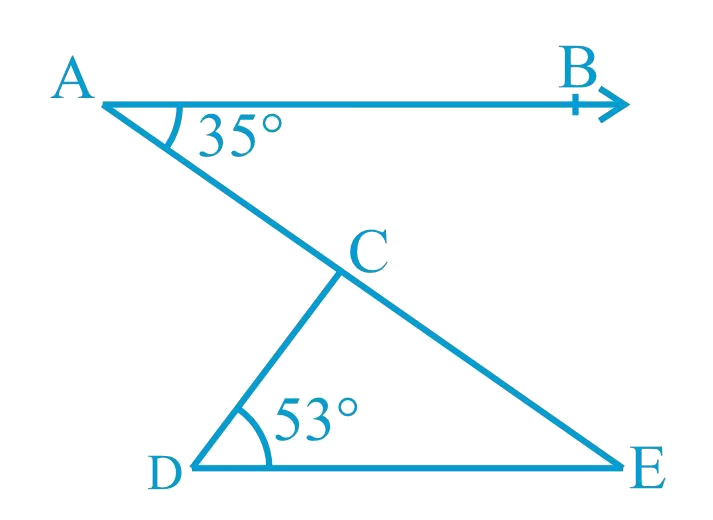# Ex.6.3 Q3 Lines and Angles Solution - NCERT Maths Class 9

Go back to  'Ex.6.3'

## Question

In Fig. below, if $$AB || DE$$, $$\angle BAC = 35^\circ$$ and $$\angle CDE = 53^\circ$$, find $$\angle DCE$$.## Text Solution

What is known?

$$AB || DE$$, $$\angle BAC = 35^\circ$$ and $$\angle CDE = 53^\circ$$

What is unknown?

$$\angle DCE$$

Reasoning:

As we know when two parallel lines are cut by a transversal, alternate interior angles formed are equal.

Angle sum property of a triangle:

Sum of the interior angles of a triangle is $$360^\circ$$.

Steps:

Given,

$$AB || DE$$ , $$\angle BAC = 35^\circ$$ and $$\angle CDE = 53^\circ$$

\begin{align}\angle DEC &= \!\angle BAC\\( \text{Alternate }&\text{interior angles})\\\\\angle DEC &= 35^\circ \end{align}

Now, in $$\Delta CDE$$

\begin{align}\angle CDE + \angle DEC + \angle DCE &= 180^\circ\quad \\( \text{Angle sum property of a}&\text{ triangle.})\\\\53^\circ + 35^\circ + \angle DCE &= 180^\circ \\\angle DCE &\!= 180^\circ \! - 88^\circ \\\angle DCE &= 92^\circ\end{align}

Learn from the best math teachers and top your exams

• Live one on one classroom and doubt clearing
• Practice worksheets in and after class for conceptual clarity
• Personalized curriculum to keep up with school